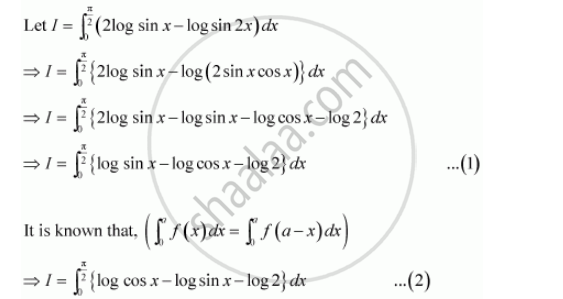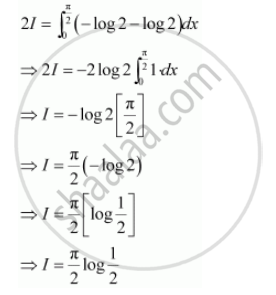Share

# By Using the Properties of Definite Integrals, Evaluate the Integrals Int_0^(Pi/2) (2log Sin X - Log in 2x)Dx - Mathematics

#### Question

By using the properties of definite integrals, evaluate the integrals

int_0^(pi/2) (2log sin x - log sin 2x)dx

#### SolutionAdding (1) and (2), we obtainIs there an error in this question or solution?

#### Video TutorialsVIEW ALL 

Solution By Using the Properties of Definite Integrals, Evaluate the Integrals Int_0^(Pi/2) (2log Sin X - Log in 2x)Dx Concept: Properties of Definite Integrals.
S